International
Tables for
Crystallography
Volume A
Space-group symmetry
Edited by Th. Hahn

International Tables for Crystallography (2006). Vol. A, ch. 8.1, pp. 724-725

## Section 8.1.6. Space groups and point groups

H. Wondratscheka*

aInstitut für Kristallographie, Universität, D-76128 Karlsruhe, Germany
Correspondence e-mail: hans.wondratschek@physik.uni-karlsruhe.de

### 8.1.6. Space groups and point groups

| top | pdf |

As mentioned in Section 8.1.3, the set of all symmetry operations of an object forms a group, the symmetry group of that object.

Definition: The symmetry group of a three-dimensional crystal pattern is called its space group. In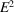, the symmetry group of a (two-dimensional) crystal pattern is called its plane group. In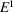, the symmetry group of a (one-dimensional) crystal pattern is called its line group. To each crystal pattern belongs an infinite set of translations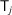which are symmetry operations of that pattern. The set of allforms a group known as the translation subgroupof the space groupof the crystal pattern. Since the commutative law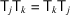holds for any two translations,is an Abelian group.

With the aid of the translation subgroup, an insight into the architecture of the space groupcan be gained.

Referred to a coordinate system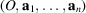, the space groupis described by the set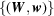of matrices W and columns w. The groupis represented by the set of elements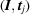, where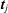are the columns of coefficients of the translation vectors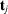of the lattice L. Let (W, w) describe an arbitrary symmetry operationof. Then, all products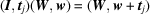for the different j have the same matrix part W. Conversely, every symmetry operationof the space group with the same matrix part W is represented in the set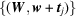. The corresponding set of symmetry operations can be denoted by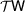. Such a set is called a right coset ofwith respect to, because the elementis the right factor in the products. Consequently, the space groupmay be decomposed into the right cosets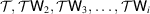, where the symmetry operations of the same column have the same matrix part W, and the symmetry operations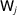differ by their matrix parts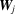. This coset decomposition ofwith respect tomay be displayed by the array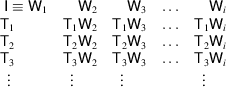Here,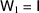is the identity operation and the elements ofform the first column, those of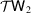the second column etc. As each column may be represented by the common matrix part W of its symmetry operations, the number i of columns, i.e. the number of cosets, is at the same time the number of different matrices W of the symmetry operations of. This number i is always finite, and is the order of the point group belonging to, as explained below. Any element of a coset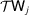may be chosen as the representative element of that coset and listed at the top of its column. Choice of a different representative element merely results in a different order of the elements of a coset, but the coset does not change its content.5

Analogously, a coset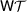is called a left coset ofwith respect to, andcan be decomposed into the left cosets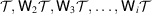. This left coset decomposition of a space group is always possible with the same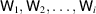as in the right coset decomposition. Moreover, both decompositions result in the same cosets, except for the order of the elements in each coset. A subgroup of a group with these properties is called a normal subgroup of the group; cf. Ledermann (1976). Thus, the translation subgroupis a normal subgroup of the space group.

The decomposition of a space group into cosets is the basis of the description of the space groups in these Tables. The symmetry operations of the space group are referred to a conventional' coordinate system (cf. Section 8.3.1) and described bymatrices. In the space-group tables as general position (cf. Section 8.3.2) for each column, a representative is listed whose coefficients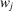obey the condition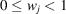. The matrix is not listed completely, however, but is given in a short-hand notation. In the expression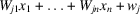, all vanishing terms and all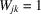are omitted, e.g.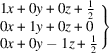is replaced by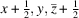. The first entry of the general position is always the identity mapping, listed as x, y, z. It represents all translations of the space group too.

As groups, some space groups are more complicated than others. Most easy to survey are the symmorphic' space groups which may be defined as follows:

Definition: A space group is called symmorphic if the coset representativescan be chosen in such a way that they leave one common point fixed.

In this case, the representative symmetry operationsof a symmorphic space group form a (finite) group. If the fixed point is chosen as the origin of the coordinate system, the column parts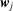of the representative symmetry operationsobey the equations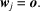Thus, for a symmorphic space group the representative symmetry operations may always be described by the special matrix–column pairs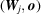.

Symmorphic space groups may be easily identified by their Hermann–Mauguin symbols because these do not contain any glide or screw operation. For example, the monoclinic space groups with the symbols P2, C2, Pm, Cm,andare symmorphic, whereas those with the symbols, Pc, Cc,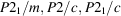andare not.

Unlike most textbooks of crystallography, in this section point groups are treated after space groups because the space group of a crystal pattern, and thus of a crystal structure, determines its point group uniquely.

The external shape (morphology) of a macroscopic crystal is formed by its faces. In order to eliminate the influence of growth conditions, the set of crystal faces is replaced by the set of face normals, i.e. by a set of vectors. Thus, the symmetry group of the macroscopic crystal is the symmetry group of the vector set of face normals. It is not the group of motions in point space, therefore, that determines the symmetry of the macroscopic crystal, but the corresponding group of linear mappings of vector space; cf. Section 8.1.2. This group of linear mappings is called the point group of the crystal. Since to each macroscopic crystal a crystal structure corresponds and, furthermore, to each crystal structure a space group, the point group of the crystal defined above is also the point group of the crystal structure and the point group of its space group.

To connect more formally the concept of point groups with that of space groups in n-dimensional space, we consider the coset decomposition of a space groupwith respect to the normal subgroup, as displayed above. We represent the right coset decomposition by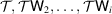and the corresponding left coset decomposition by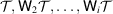. Ifis referred to a coordinate system, the symmetry operations ofare described by matrices W and columns w. As a result of the one-to-one correspondence between the i cosets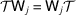and the i matrices, the cosets may alternatively be represented by the matrices. These matrices form a group of (finite) order i, and they describe linear mappings of the vector spaceconnected with; cf. Section 8.1.2. This group (of order i) of linear mappings is the point groupof the space group, introduced above.

The difference between symmetry in point space and that in vector space may be exemplified again by means of some monoclinic space groups. Referred to conventional coordinate systems, space groups Pm, Pc, Cm and Cc have the samematricesof their symmetry operations. Thus, the point groups of all these space groups are of the same type m. The space groups themselves, however, show a rather different behaviour. In Pm and Cm reflections occur, whereas in Pc and Cc only glide reflections are present.

Remark: The usage of the term point group' in connection with space groups is rather unfortunate as the point group of a space group is not a group of motions of point space but a group acting on vector space. As a consequence, the point group of a space group may contain operations which do not occur in the space group at all. This is apparent from the example of monoclinic space groups above. Nevertheless, the term point group of a space group' is used here for historical reasons. A more adequate term would be vector point group' of a space group or a crystal. It must be mentioned that the term point group' is also used for the `site-symmetry group', defined in Section 8.3.2. Site-symmetry groups are groups acting on point space.

It is of historic interest that the 32 types of three-dimensional crystallographic point groups were determined more than 50 years before the 230 (or 219) types of space group were known. The physical methods of the 19th century, e.g. the determination of the symmetry of the external shape or of tensor properties of a crystal, were essentially methods of determining the point group, not the space group of the crystal.

### References

Ledermann, W. (1976). Introduction to group theory. London: Longman.Google Scholar
Souvignier, B. (2003). Enantiomorphism of crystallographic groups in higher dimensions with results in dimensions up to 6. Acta Cryst. A59, 210–220.Google Scholar